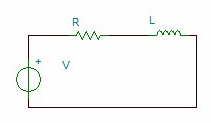Introduction To Electric Circuits - 9 Edition - Chapter 7.5 - Problem P7.5-2
Register Now

Join StudySoup

Get Full Access to Introduction To Electric Circuits - 9 Edition - Chapter 7.5 - Problem P7.5-29781118477502

# The model of an electric motor consists of a series combination of a resistor and

Introduction to Electric Circuits | 9th Edition

Problem P7.5-2

The model of an electric motor consists of a series combination of a resistor and inductor. A current i t 4tet A flows through the series combination of a 10-V resistor and 0.1-H inductor. Find the voltage across the combination.

Accepted Solution
Step-by-Step Solution:

Step 1 of 3###### Chapter 7.5, Problem P7.5-2 is Solved

Step 2 of 3

Step 3 of 3

Unlock Textbook Solution